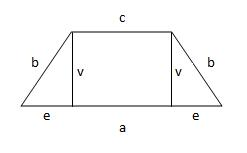We cut two triangles from the rectangular plate so that the resulting quadrilateral-trapezoid with the same arm lengths has an area of 32 cm2, and one of its bases is twice as long as the other. What is the area of the two triangles that make up the waste?

S =  10.6667 cm2

### Step-by-step explanation:Did you find an error or inaccuracy? Feel free to write us. Thank you!

Tips for related online calculators
Do you have a linear equation or system of equations and looking for its solution? Or do you have a quadratic equation?